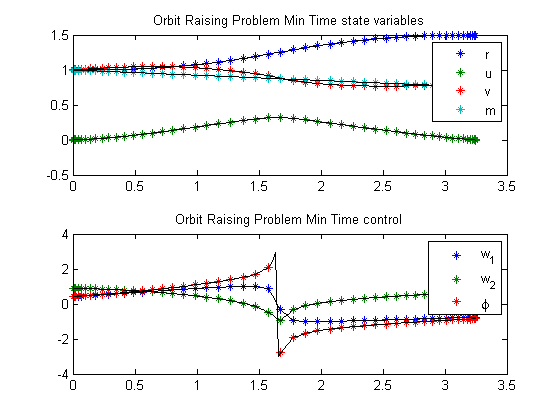79  Orbit Raising Minimum Time

79.1  Problem description

Minimize:

 J = tf

subject to the dynamic constraints

 dr dt
= u
 du dt
=
 v2 r
 mmu r2
+T*
 w1 m

 dv dt
= −u*
 v r
+T*
 w2 m

 dm dt
= −
 T g0*ISP

the boundary conditions

 r(t0) = 1
 u(t0) = 1
 u(tf) = 0
v(t0) = (
 mmu r(t0)
)0.5
v(tf) = (
 mmu r(tf)
)0.5
 m(t0) = 1

where w1 = sin(phi) and w2 = cos(phi)

At t_f, r and m are free.

Reference: 

79.2  Problem setup

mmu     = 1;
t_f    = 3.32;  m_0    = 1;            r_0    = 1;   u_0    = 0;
u_f    = 0;     v_0    = sqrt(mmu/r_0); rmin   = 0.9; rmax   = 5;
umin   = -5;    umax   = 5;            vmin   = -5;  vmax   = 5;
mmax   = m_0;   mmin   = 0.1;          tf_min = 0.5; tf_max = 10;
r_f     = 1.5;

T  = 0.1405;
Ve = 1.8758;

toms t t_f
p1 = tomPhase('p1', t, 0, t_f, 50);
setPhase(p1);

tomStates r u v m

% The problem becomes less nonlinear if w1 and w2 are control variables
% (with the constraints w1^2+w2^2==1) than if phi is the control varialbe
% (with w1 and w2 being nonlinear functions of phi).
tomControls w1 w2
phi = atan2(w1,w2);

% Initial guess
x0 = {t_f == 3.32
icollocate({
r == r_0+(r_f-r_0)*t/t_f
u == 0.1
v == v_0
m == m_0-(T/Ve)*t})
collocate({
w1 == -0.7*sign(t-t_f/2)
w2 == 0.4
})};

% Boundary constraints
cbnd = {initial({
r == r_0
u == u_0
v == v_0
m == m_0
})
final({
r == r_f
u == u_f
v == sqrt(mmu/r)})};

% Box constraints
cbox = {0.5 <= t_f <= 10
rmin <= icollocate(r) <= rmax
umin <= icollocate(u) <= umax
vmin <= icollocate(v) <= vmax
};

% ODEs and path constraints
ceq = collocate({
dot(r) == u
dot(u) == v^2/r-mmu/r^2+T*w1/m
dot(v) == -u*v/r+T*w2/m
dot(m) == -T/Ve
w1^2+w2^2 == 1
});

% Objective
objective = t_f;

79.3  Solve the problem

options = struct;
options.name = 'Orbit Raising Problem Min Time';
options.scale = 'manual'; % Auto-scaling is not really needed as all variables are already reasonably scaled.
solution = ezsolve(objective, {cbox, cbnd, ceq}, x0, options);
Problem type appears to be: lpcon
Starting numeric solver
===== * * * =================================================================== * * *
TOMLAB - Tomlab Optimization Inc. Development license  999001. Valid to 2011-02-05
=====================================================================================
Problem: ---  1: Orbit Raising Problem Min Time  f_k       3.248079535630944200
sum(|constr|)      0.000032253415551923
f(x_k) + sum(|constr|)      3.248111789046496300
f(x_0)      3.319999999999999800

Solver: snopt.  EXIT=0.  INFORM=1.
SNOPT 7.2-5 NLP code
Optimality conditions satisfied

FuncEv    1 ConstrEv   68 ConJacEv   68 Iter   35 MinorIter  283
CPU time: 2.140625 sec. Elapsed time: 1.187000 sec.

79.4  Plot result

subplot(2,1,1)
ezplot([r u v m]);
legend('r','u','v','m');
title('Orbit Raising Problem Min Time state variables');

subplot(2,1,2)
ezplot([w1 w2 phi])
legend('w_1', 'w_2', '\phi');
title('Orbit Raising Problem Min Time control');